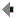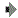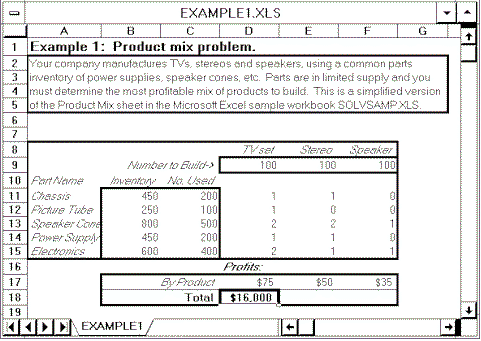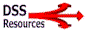Book Contents

Ch. 9
Building Model-Driven Decision Support SystemsNetwork and Optimization Models

Project planning and control, location, allocation, distribution and transportation problems can often be formulated using network and optimization models. We can use the models to determine: Where is the best location for an operation? How big should facilities be? What resources are needed? Are there shortages? Networks can define many relationships and network problems are most often solved using optimization models.

For example, we can define and analyze a network of project activities using project management software. Project management is a popular category of off-the-shelf decision support software. The best selling package is Microsoft Project. It is a powerful application that you can use to efficiently plan, manage, and communicate project information. Project managers can enter actual costs for tasks and assignments. Check http://www.microsoft.com/ office/project. While many computer users are familiar with project management software not everyone realizes it is based on network flow models. These models are specially structured linear programming problems.

DSS Analysts can define other networks. For example, one can develop a network of possible airline routes and schedules and compare costs. A set of routes or paths can be analyzed using a number of heuristic or quantitative tools. It has been estimated that 70% of all linear programming applications are network flow problems or have a substantial network structure. In addition to project management and aircraft routing, applications include: production planning and aggregate scheduling, personnel planning and scheduling, land use allocation, classroom scheduling, plant location, multinational cash flow management, and integrated production-inventory-distribution. Often a network model can be depicted as a set of nodes and arcs. Nodes may be sources of product and or demanders of product. Units of product can move from one node to another across arcs. For more information check Richard Barr’s Survey of Network Models at www.seas.smu.edu/~barr/ip/ch0/ch0.html.

Linear programming is the most widely known technique in a family of tools called mathematical programming. There are many possible uses of mathematical programming, especially of linear programming, in organizations. Many books have been written for courses in Management Science, Quantitative Analysis and Operations Research. Managers and DSS specialists are usually not experts in using optimization or simulation tools. Small-scale optimization DSS can be built using a spreadsheet program like Microsoft Excel. An on-line Web-based tutorial on Using MS Excel Solver for Spreadsheet Optimization is at the Frontline Systems Web site (http://frontsys.com/).

Linear programming attempts to either maximize or minimize the values of an objective function. A solver program can be used for both equation-solving or goal-seeking and constrained optimization using linear programming, nonlinear programming, and integer programming methods.

Users of a Model-Driven DSS based on a linear programming model can find input values that satisfy a set of simultaneous equations and inequalities. When a user does this there is usually more than one satisfactory set of input values. So a Solver can find the "best" set of input values that maximizes or minimizes some other calculated formula that you specify. This process is called constrained optimization; the equations or inequalities are called constraints. Every linear programming problem is composed of six elements:

Decision Variables. These variables are unknown values that are searched for by applying the model. Usually decision variables are designated by X1, X2, …, XN .

Objective Function. This is a mathematical expression that shows the linear relationship between the decision variables and the single goal that is the focus of the model. The objective function is a measure of goal attainment. Examples of such goals are total profit, total cost, and market share.

Coefficients of the Objective Function. The coefficients of the variables in the objective function are called the profit or cost coefficients. They express the rate at which the value of the objective function increases or decreases by including in the solution one unit of each of the decision variables.

Constraints. The maximization or minimization is performed subject to a set of constraints. Therefore, linear programming can be defined as a constrained optimization problem. These constraints are expressed in the form of linear inequalities or, sometimes, equalities. They reflect the fact that resources are limited or they specify some requirements.

Input-Output (Technology) Coefficients. The coefficients of the constraints' variables are called the input-output coefficients. They indicate the rate at which a given resource is depleted or utilized.

Capacities. The capacities or availability of the various resources is usually expressed as some upper or lower limit. When a problem is formulated the capacities also express minimum requirements.Figure 9.5 An Example Optimization Model developed in Microsoft Excel

In Figure 9.5 the decision variables are the quantities of TVs, stereos and speakers to build. The objective function is to maximize total profits. The constraints are from the parts inventory. Managers should be able to build a simple Model-Driven DSS like Figure 9.5 in Excel. A support person may need to help in conceptualizing the problem or in testing the end user application. For more information on Optimization and Linear Programming check Harvey Greenberg's excellent Mathematical Programming glossary at URL http://www.cudenver.edu/~hgreenbe/glossary/intro.html and Michael Trick's Operations Research Page at URL http://mat.gsia.cmu.edu/index.html.

Most often optimization models are included in a DSS to assist in resource allocation. Managers are often required to allocate productive resources like raw materials, people, money or time, which can be used in a variety of different ways. The problem is to determine the best way to use the resources. Managers need to determine what "best" means, but usually it implies maximizing profits, minimizing costs, maximizing quality or minimizing the risk of failure (cf., http://frontsys.com/tutorial.htm).DSSResources.COMsm is maintained and all its pages are copyrighted (c) 1995-2002 by D. J. Power (see home page). Please contact power@dssresources.com. This page was last modified Wednesday, May 30, 2007. See disclaimer and privacy statement.